Abel summation method

(diff) ← Older revision | Latest revision (diff) | Newer revision → (diff)

One of the methods for the summation of series of numbers. The seriescan be summed by the Abel method (-method) to the numberif, for any real,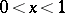, the series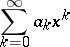is convergent and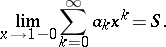This summation method can already be found in the works of L. Euler and G. Leibniz. The name "Abel summation method" originates from the Abel theorem on the continuity of power series. The Abel summation method belongs to the class of totally regular summation methods and is more powerful than the entire set of Cesàro summation methods. The Abel summation method is used in conjunction with Tauberian theorems to demonstrate the convergence of a series.

A closely related summation method is the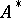-method. Letbe a complex number,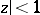; the series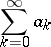is summed by the-method to the numberifwhere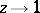along any path not tangent to the unit circle.